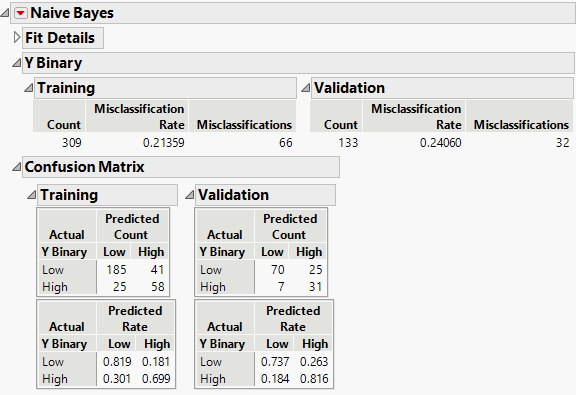Publication date: 03/23/2021

#The Naive Bayes Report

After you click OK in the launch window, the Naive Bayes report appears. By default, the Naive Bayes report contains reports for fit details, the response column, the Confusion Matrix Report, and the ROC and Lift curves.

Figure 8.7 Naive Bayes Report##Fit Details Report

The Fit Details report shows various measures of fit for the model for the Training set, and for the Validation and Test sets if they are specified. The Measure column lists the different fit statistics and the Definition column shows the formulas for the corresponding fit statistics. See Measures of Fit for Categorical Responses. By default, the Fit Details report in the Naive Bayes report window is closed.

##Response Column Report

The response column report shows performance statistics for the naive Bayes classification in a summary table for the Training set, and the Validation and Test sets if they are specified. The summary tables contain the following columns:

Count

The number of observations in the set corresponding to the table (Training, Validation, or Test set).

Misclassification Rate

Proportion of observations in the corresponding set that are misclassified by the model. This is calculated as Misclassifications divided by Count.

Misclassifications

The number of observations in the corresponding set that are classified incorrectly.

##Confusion Matrix Report

The Confusion Matrix Report shows a confusion matrix and a confusion rates matrix for the Training set, and for the Validation and Test sets if they are specified. A confusion matrix is a two-way classification of actual and predicted responses. A confusion rates matrix is equal to the confusion matrix, with the numbers divided by the row totals.

##ROC Curves

The report displays the Receiver Operating Characteristic (ROC) curve for the Training set, and for the Validation and Test sets if they are specified. The ROC curve measures the ability of the fitted probabilities to classify response levels correctly. The further the curve from the diagonal, the better the fit. An introduction to ROC curves is found in ROC Curves in Basic Analysis.

See ROC Curve for more information about ROC curves.

Want more information? Have questions? Get answers in the JMP User Community (community.jmp.com).